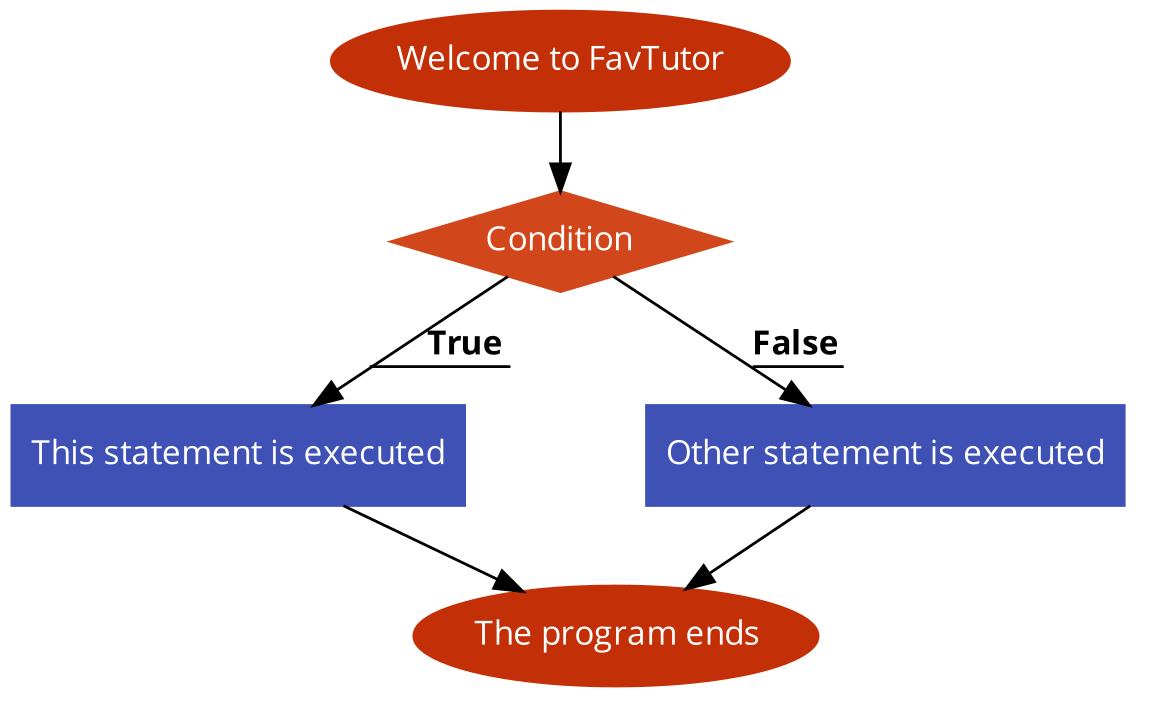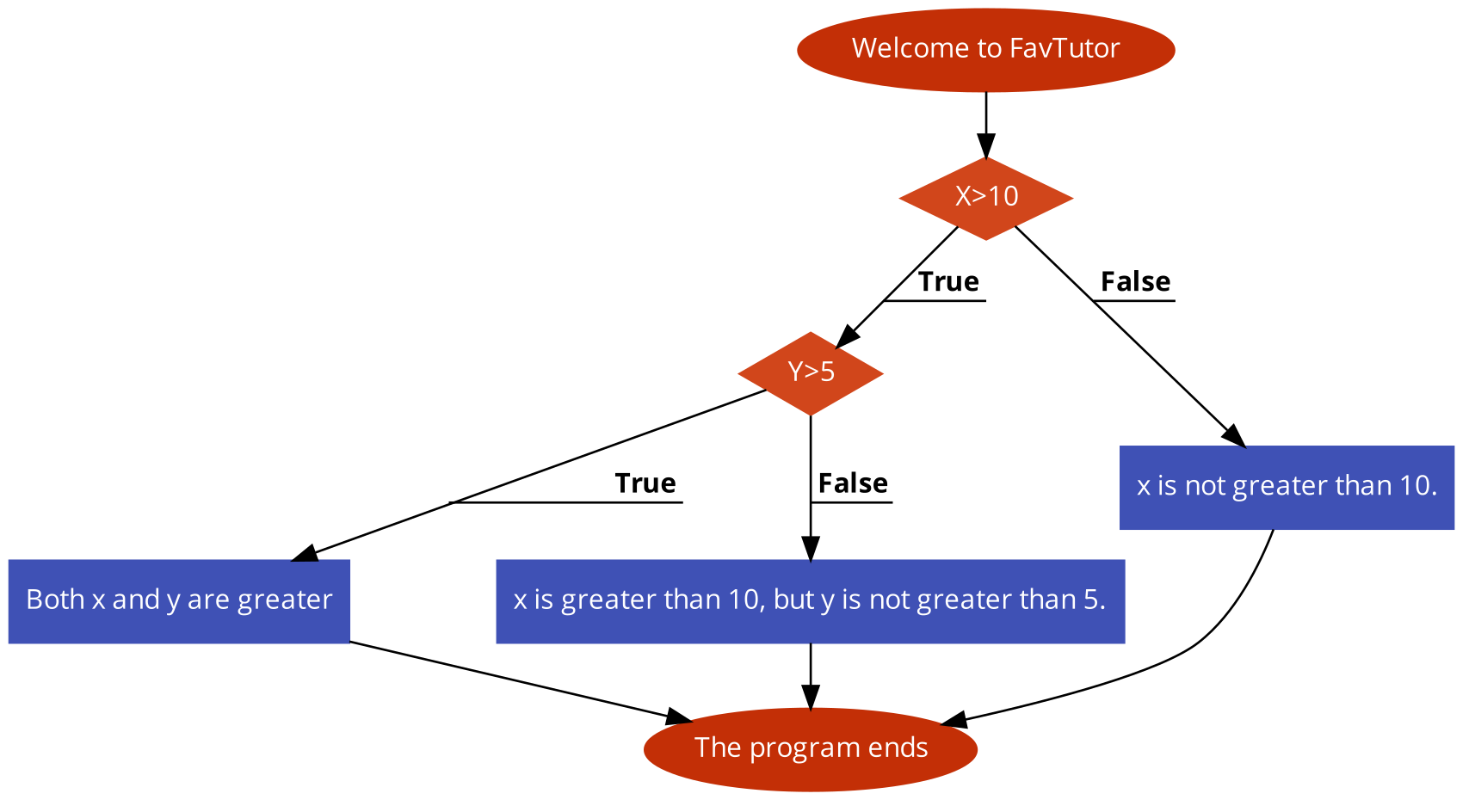# ifelse() function in R (with Code)

If-else statements are fundamental constructs in programming languages, including R. These statements allow you to make decisions and control the flow of your code based on certain conditions. In this article, we will dive deep into the world of if-else statements in R, exploring their syntax, usage, and best practices.

## Understanding the Basics of If-Else Statements

At its core, an if-else statement in R is used to execute different blocks of code based on a condition's evaluation. The basic syntax of an if-else statement looks like this:

```if (condition) {
# Code to execute if the condition is TRUE
} else {
# Code to execute if the condition is FALSE
}
```

Here's a breakdown of how this works:

• `condition` represents a logical expression that evaluates to either TRUE or FALSE.
• If `condition` is TRUE, the code block inside the if block is executed.
• If `condition` is FALSE, the code block inside the else block is executed.Let's look at some practical examples to understand how if-else statements work in real-world scenarios.

### Example 1: Basic if-else Statement

```x <- 10

if (x > 5) {
print("x is greater than 5")
} else {
print("x is not greater than 5")
} ```

In this example, since x is indeed greater than 5, the message "x is greater than 5" will be printed to the console.

Output:

```x is greater than 5
```

### Example 2: Handling Multiple Conditions

You can also use `else if` to handle multiple conditions:

```x <- 10

if (x > 15) {
print("x is greater than 15")
} else if (x > 5) {
print("x is greater than 5 but not greater than 15")
} else {
print("x is not greater than 5")
}
```

Here, the code will execute the second block because the first condition is FALSE, but the second condition (x > 5) is TRUE.

Output:

```x is greater than 5 but not greater than 15
```

Whenever a condition is met in the if-else statements, the program executes statements and operations under that condition and exits the control flow.

## Deeper Dive into If-Else Statements

Now that we are acquainted with the basics of if-else, let us now understand other types of If-Else statements in depth.

### Vectorized If-Else: ifelse()

In R, there's a vectorized version of if-else called ifelse(). This function is particularly useful when dealing with vectors or data frames. Its syntax is as follows:

```result_vector <- ifelse(condition, true_value, false_value)
```

Here's an example using ifelse():

```grades <- c(85, 92, 78, 65, 70)

pass_fail <- ifelse(grades >= 70, "Pass", "Fail")

print(pass_fail)
```

Output:

```(Pass, Pass, Pass, Fail, Pass)
```

### Nested If-Else Statements

You can nest if-else statements within one another to handle more complex scenarios. This allows you to create decision trees with multiple branches.Here's an example:

```x <- 15
y <- 10

if (x > 10) {
if (y > 5) {
print("Both x and y are greater than their respective thresholds.")
} else {
print("x is greater than 10, but y is not greater than 5.")
}
} else {
print("x is not greater than 10.")
}
```

Output:

```Both x and y are greater than their respective thresholds
```

### The switch() Function

The switch() function is another way to handle multiple conditions more elegantly. It selects a value from a set of choices based on an integer or character expression. Here's an example:

```day <- 3

day_name <- switch(day,
"Sunday",
"Monday",
"Tuesday",
"Wednesday",
"Thursday",
"Friday",
"Saturday")

print(paste("Today is", day_name))
```

Output:

```Today is Tuesday
```

The switch() function here assigns the appropriate day name based on the value of the day.

## Best Practices and Tips

1. Use ifelse() for Vectorized Operations: When working with vectors or data frames, prefer ifelse() for efficiency and simplicity.

2. Indentation and Readability: Proper indentation and clear formatting make your code more readable. It's essential to maintain a consistent coding style.

3. Avoid Overly Nested Statements: While nested if-else statements are powerful, don't go overboard with nesting, as it can make your code hard to follow. Consider breaking down complex logic into smaller, more manageable functions.

4. Use switch() for Multiple Choices: When dealing with multiple choices based on a single expression, switch() is a clean and efficient option.

5. Testing: Always test your if-else statements with various inputs to ensure they behave as expected. Use test cases to cover different scenarios.

## Conclusion

If-else statements are a fundamental building block of any programming language. They provide the means to make decisions and control the flow of your code. Whether you're working with simple conditions or complex decision trees, mastering if-else statements in R is essential for becoming a proficient R programmer.

### FavTutor - 24x7 Live Coding Help from Expert Tutors!